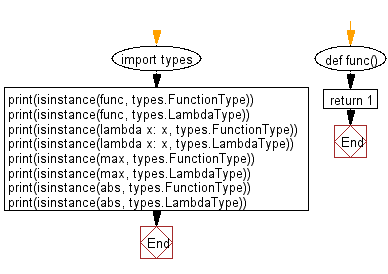﻿ Python: Check if a function is a user-defined function or not - w3resource# Python: Check if a function is a user-defined function or not

## Python module: Exercise-1 with Solution

Write a Python program to check if a function is a user-defined function or not. Use types.FunctionType, types.LambdaType()

Sample Solution:

Python Code:

``````import types
def func():
return 1

print(isinstance(func, types.FunctionType))
print(isinstance(func, types.LambdaType))
print(isinstance(lambda x: x, types.FunctionType))
print(isinstance(lambda x: x, types.LambdaType))
print(isinstance(max, types.FunctionType))
print(isinstance(max, types.LambdaType))
print(isinstance(abs, types.FunctionType))
print(isinstance(abs, types.LambdaType))
```
```

Sample Output:

```True
True
True
True
False
False
False
False
```

Flowchart:## Visualize Python code execution:

The following tool visualize what the computer is doing step-by-step as it executes the said program:

Python Code Editor:

Have another way to solve this solution? Contribute your code (and comments) through Disqus.

What is the difficulty level of this exercise?

Test your Python skills with w3resource's quiz

﻿

## Python: Tips of the Day

For-else construct useful when searched for something and find it:

```# For example assume that I need to search through a list and process each item until a flag item is found and
# then stop processing. If the flag item is missing then an exception needs to be raised.

for i in mylist:
if i == theflag:
break
process(i)
else:
raise ValueError("List argument missing terminal flag.")
```